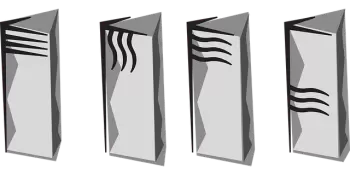# Characteristics of Prisms: Definition, Examples and TypesA prism is a polyhedron whose two bases are congruent (equal) polygons lying in parallel planes. The lateral faces are flat faces that form parallelograms with standard sides with these polygons. These parallelograms are called the side faces of the prism, and the remaining two polygons are called their bases.

The differences between a prism and a pyramid are as follows:

• First, the pyramid has only one base.

• The lateral faces of the pyramids are isosceles triangles. On the contrary, in the prisms, the lateral faces are parallelograms.

• Pyramids have fewer faces, edges, and vertices.

## Characteristics of the Prisms

• The two bases of a prism are made up of two identical polygons.

• The lateral faces of the geometric body are parallelograms, rectangular faces.

• The lateral edges are equal to each other and parallel.

• The volume of a prism is obtained by multiplying the area of ​​the base by the total height of the polyhedron:

• The area of ​​the total surface of the polyhedron is equal to the sum of the area of ​​all its faces, that is, the sum of the lateral surfaces and twice the area of ​​the base.

• The lateral surface area of ​​a prism can be calculated by the formula S=P·l, where “P” is the perimeter of the perpendicular section, “l” is the length of the lateral rib.

• The lateral surface of a right prism S=P·h, where “P” is the perimeter of the base and “h” is the height of the geometric figure.

• All lateral edges are perpendicular to any cross-section parallel to the base of the 3D shape.

• The angles of a perpendicular section are the linear angles of the dihedral angles at the corresponding side edges.

• All lateral faces are perpendicular to any perpendicular cross-section applied to the element.

• The center of the bases coincides with the axis of symmetry.

## Examples of Prisms

The polygon at the base determines the name of the prism. Some examples of prominent prisms are:

### Triangular Prism

The triangular prism is a polyhedron with two triangular bases joined by three side faces that are parallelograms.

A triangular prism cannot be a regular polyhedron because not all of its faces are regular polygons (with equal sides and interior angles) and identical.

A quadrangular prism is a three-dimensional shape formed by two equal and parallel quadrilaterals called bases and by four lateral faces that are parallelograms.

The regular quadrangular prism has two squares as bases. Its lateral faces are equal rectangles.

The irregular quadrangular prism has two quadrilaterals that are not squares as bases. Five cases can occur: their bases can be rectangles, rhombuses, rhomboids, trapezoids, or trapezoids.

A particular case is a square prism whose bases are squares.

### Pentagonal Prism

A pentagonal prism is a shape obtained by two equal and parallel pentagons called bases and by five lateral faces that are parallelograms.

These are the most significant features of the pentagonal prism:

• Bases: are two parallel and equal pentagons.

• Faces are the five parallelograms of the lateral faces and the two bases; therefore, they have seven faces.

• Height: distance between the two bases of the prism. In the case of a right prism, the length of the height h and the edges of the lateral faces coincide.

• It has ten vertices which are the points where three faces of the prism meet.

• Edges: segments where two faces of the prism meet.
By Euler's theorem, the number of edges can be known by knowing the number of faces and vertices. Therefore, the number of edges of a pentagonal prism is 15.

### Hexagonal Prism

A hexagonal prism is a polyhedron whose surface is formed by two equal and parallel hexagons called bases and by six lateral faces that are parallelograms.

We can see the following properties in a hexagonal prism:

• Its bases are two parallel equal hexagons.

• It has six lateral faces, and all of them are parallelograms.

• A hexagonal prism has twelve vertices.

• It has 18 edges, according to Euler's theorem.

## Types of Prisms

A right prism is a prism whose lateral edges and lateral faces are regular polygons perpendicular to the bases. This definition of a prism implies that all lateral faces are rectangles.

A regular prism is a right prism whose two bases are made up of regular polygons. The lateral faces of this type of prism are identical rectangles.

The irregular prism is a polyhedron formed by two parallel and equal faces, which are irregular polygons, and also has lateral faces that are parallelograms.

Oblique prisms have edges that are not perpendicular to the base plane.

Author:

Published: May 29, 2022
Last review: May 29, 2022